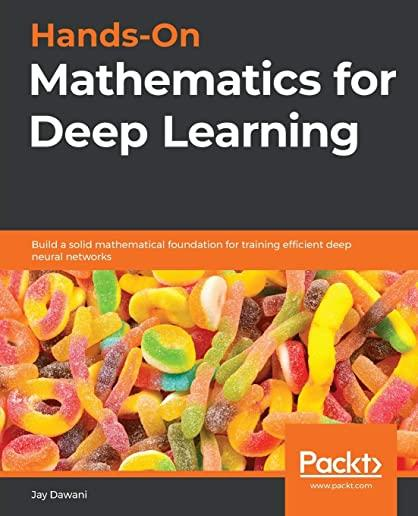### Hands-On Mathematics for Deep Learning: Build a solid mathematical foundation for training efficient deep neural networks### Huge savings for students

Each student receives a 50% discount off of most books in the HSG Book Store. During class, please ask the instructor about purchase details.
 List Price: \$39.99 Price: \$20.00 You Save: \$20.00
7

A comprehensive guide to getting well-versed with the mathematical techniques for building modern deep learning architectures

Key Features

• Understand linear algebra, calculus, gradient algorithms, and other concepts essential for training deep neural networks
• Learn the mathematical concepts needed to understand how deep learning models function
• Use deep learning for solving problems related to vision, image, text, and sequence applications

Book Description

Most programmers and data scientists struggle with mathematics, having either overlooked or forgotten core mathematical concepts. This book uses Python libraries to help you understand the math required to build deep learning (DL) models.

You'll begin by learning about core mathematical and modern computational techniques used to design and implement DL algorithms. This book will cover essential topics, such as linear algebra, eigenvalues and eigenvectors, the singular value decomposition concept, and gradient algorithms, to help you understand how to train deep neural networks. Later chapters focus on important neural networks, such as the linear neural network and multilayer perceptrons, with a primary focus on helping you learn how each model works. As you advance, you will delve into the math used for regularization, multi-layered DL, forward propagation, optimization, and backpropagation techniques to understand what it takes to build full-fledged DL models. Finally, you'll explore CNN, recurrent neural network (RNN), and GAN models and their application.

By the end of this book, you'll have built a strong foundation in neural networks and DL mathematical concepts, which will help you to confidently research and build custom models in DL.

What you will learn

• Understand the key mathematical concepts for building neural network models
• Discover core multivariable calculus concepts
• Improve the performance of deep learning models using optimization techniques